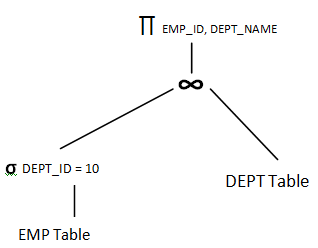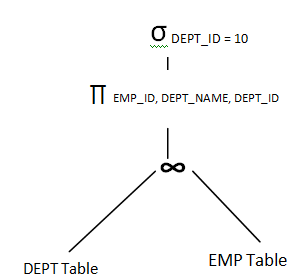# Transformation of Relational Expressions in DBMS

## Introduction

When a query is submitted to the database, it is responsibility of database to determine algorithm to evaluate the query. The DBMS has to decide a low cost algorithm to evaluate the query and then optimal path to evaluate the query. The query may be simple or complex. Depending on the cost it has to pick better execution path. This is called query optimization.
There are various factors affecting the performance of the query like number of records in the table, number of blocks allocated to each table, number of records in each block, size of the record, duplicate records, height of B+ tree, constraints and indexes etc. Let us see how to select a better performing query.

## Transformation of Relational Expressions

When a SQL query is submitted to DB, it can be evaluated in number of ways. For example, consider the below case:

```SELECT EMP_ID, DEPT_NAME
FROM EMP, DEPT
WHERE EMP.DEPT_ID = DEPT.DEPT_ID
AND EMP.DEPT_ID = 10;```

Above query selects the EMP_ID and DEPT_NAME from EMP and DEPT table for DEPT_ID = 10. But when it is given to the DBMS, it divides the query into tokens and sees how it can be put together so that performance will be better. This is the duty of query optimizer. But altering the order of tokens in the query should not change the result. In either way it should give same result. Order of records can change and are least important. This is called equivalent query. There is set of rules to put tokens in the query. This is called equivalence rule.

## Above query can be broken down by the DBMS in either ways below :

• Select the records of EMP with DEPT_ID = 10 first then join them with DEPT table to get all matching records of EMP and DEPT. Then select only the columns EMP_ID and DEPT_NAME to display the result.
• Select all matching records from EMP and DEPT, from which filter on DEPT_ID = 10 and select only EMP_ID and DEPT_NAME to display.

Both the steps above are same irrespective of how it is performed. Hence both are called equivalent query. These are not written in SQL, but using relational algebra, graph or tree.

∏ EMP_ID, DEPT_NAME (σ DEPT_ID = 10 (EMP ∞DEPT))

or

σ DEPT_ID = 10 (∏ EMP_ID, DEPT_NAME, DEPT_ID (EMP ∞DEPT))Above relational algebra and tree shows how DBMS depicts the query inside it. But the cost of both of them may vary.  This is because the number of records in each step changes depending on the join and filters we use, and the algorithms used to evaluate them. For example we may have huge number of records in second case tree above to filter. But in the first case we are filtering the record first; hence number of records to join would have been reduced. This makes lots of difference and query optimizer calculates this difference and selects the optimal tree for query evaluation.

Translate »